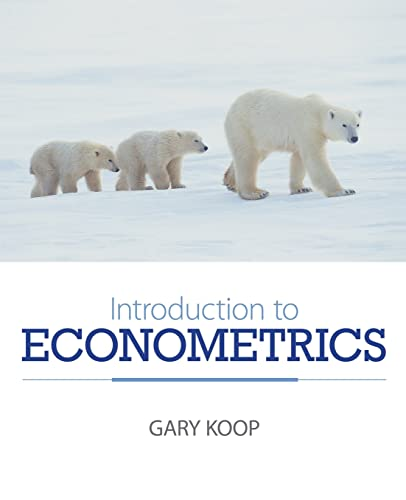Free Shipping on all orders in Australia
Over 7 million books in stock
We aim to be carbon neutral by 2022# Introduction to Econometrics by Gary Koop

Condition - Like New
\$88.99
Only 3 left

## Summary

Introduction to Econometric Modelling provides an introduction to econometrics for undergraduate students. In this book, Gary Koop provides a broader set of models than is offered in existing textbooks and places greater focus on models (e.g. the regression model) than the methods that are used to analyze the models.

## Introduction to Econometrics Summary

### Introduction to Econometrics by Gary Koop

Introduction to Econometrics has been written as a core textbook for a first course in econometrics taken by undergraduate or graduate students. It is intended for students taking a single course in econometrics with a view towards doing practical data work. It will also be highly useful for students interested in understanding the basics of econometric theory with a view towards future study of advanced econometrics. To achieve this end, it has a practical emphasis, showing how a wide variety of models can be used with the types of data sets commonly used by economists. However, it also has enough discussion of the underlying econometric theory to give the student a knowledge of the statistical tools used in advanced econometrics courses. Key Features: * A non-technical summary of the basic tools of econometrics is given in chapters 1 and 2, which allows the reader to quickly start empirical work. * The foundation offered in the first two chapters makes the theoretical econometric material, which begins in chapter 3, more accessible. * Provides a good balance between econometric theory and empirical applications. * Discusses a wide range of models used by applied economists including many variants of the regression model (with extensions for panel data), time series models (including a discussion of unit roots and cointegration) and qualitative choice models (probit and logit). An extensive collection of web-based supplementary materials is provided for this title, including: data sets, problem sheets with worked through answers, empirical projects, sample exercises with answers, and slides for lecturers. URL: www.wileyeurope.com/college/koop

## Introduction to Econometrics Reviews

"An introductory text offering econometric methodology for quantifying and managing this variety of risk, illustrated by empirical examples." ( Times Higher Education Supplement , Thursday 28th February)

## About Gary Koop

Gary Koop is Professor of Economics at the University of Strathclyde. Gary has published numerous articles econometrics in journals such as the Journal of Econometrics and Journal of Applied Econometrics . Gary has taught econometrics for many years and is the author of following textbooks, all published by John Wiley & Sons Ltd: Analysis of Economic Data 2ed, Analysis of Financial Data and Bayesian Econometrics

## Table of Contents

Preface. Chapter 1: An Overview of Econometrics. 1.1 The Importance of Econometrics. 1.2 Types of Economic Data. 1.3 Working with Data: Graphical Methods. 1.4 Working with Data: Descriptive Statistics and Correlation. 1.5 Chapter Summary. Exercises. Chapter 2: A Non-technical Introduction to Regression. 2.1 Introduction. 2.2 The Simple Regression Model. 2.3 The Multiple Regression Model. 2.4 Chapter Summary. Exercises. Chapter 3: The Econometrics of the Simple Regression Model. 3.1 Introduction. 3.2 A Review of Basic Concepts in Probability in the Context of the Regression Model. 3.3 The Classical Assumptions for the Regression Model. 3.4 Properties of the Ordinary Least Squares Estimator of beta. 3.5 Deriving a Confidence Interval for beta. 3.6 Hypothesis Tests about beta. 3.7 Modifications to Statistical Procedures when sigma 2 is Unknown. 3.8 Chapter Summary. Exercises. Appendix 1: Proof of the Gauss-Markov theorem. Appendix 2: Using a Asymototic Theory in the Simple Regression Model. Chapter 4: The Econometrics of the Multiple Regression Model. 4.1 Introduction. 4.2 Basic Results for the Multiple Regression Model. 4.3 Issues Relating to the Choice of Explanatory Variables. 4.4 Hypothesis Testing in the Multiple Regression Model. 4.5 Choice of Functional Form in the Multiple Regression Model. 4.6 Chapter Summary. Exercises. Appendix: Wald and Lagrange multiplier tests. Chapter 5: The Multiple Regression Model: Freeing up Classical Assumptions. 5.1 Introduction. 5.2 Basic Theoretical Results. 5.3 Heteroskedasticity. 5.4 The Regression Model with Autocorrelated Errors. 5.5 The Instrumental Variables Estimator. 5.6 Chapter Summary. Exercises. Appendix: Asymptotic Results for the OLS and Instrumental variables Estimators. Chapter 6: Univariate Time Series Analysis. 6.1 Introduction. 6.2 Time Series Notation. 6.3 Trends in Time Series Variables. 6.4 The Autocorrelation Function. 6.5 The Autoregressive Model. 6.6 Defining Stationarity. 6.7 Modelling Volatility. 6.8 Chapter Summary. Exercises. Appendix: MA and ARMA Models. Chapter 7: Regression with Time Series Variables. 7.1 Introduction. 7.2 Time Series Regression when X and Y are Stationary. 7.3 Time Series Regression When Y and X have Unit Roots. 7.4 Time Series Regression when Y and X have Unit Roots but are NOT Cointegrated. 7.5 Granger Causality. 7.6 Vector Autoregressions. 7.7 Chapter Summary. Exercises. Appendix: The Theory of Forecasting. Chapter 8: Models for Panel Data. 8.1 Introduction. 8.2 The Pooled Model. 8.3 Individual Effects Models. 8.4 Chapter Summary. Exercises. Chapter 9: Qualitative Choice and Limited Dependent Variable Models. 9.1 Introduction. 9.2 Qualitative Choice Models. 9.3 Limited Dependent Variable Models. 9.4 Chapter Summary. Exercises. Chapter 10: Bayesian Econometrics. 10.1 An Overview of Bayesian Econometrics. 10.2 The Normal Linear Regression Model with Natural Conjugate Prior and a Single Explanatory Variable. 10.3 Chapter Summary. 10.4 Exercises. Appendix: Bayesian Analysis of the Simple Regression Model with Unknown Variance. Appendix A; Mathematical Basics. Appendix B: Probability Basics. Appendix C: Basic Concepts in Asymptotic Theory. Appendix D: Writing an Empirical Project. Tables. Bibliography. Index.

## Additional information

GOR010912982
Introduction to Econometrics by Gary Koop
Used - Like New
Paperback
John Wiley & Sons Inc
2007-11-23
384
0470032707
9780470032701
N/A
Book picture is for illustrative purposes only, actual binding, cover or edition may vary.
The book has been read, but looks new. The book cover has no visible wear, and the dust jacket is included if applicable. No missing or damaged pages, no tears, possible very minimal creasing, no underlining or highlighting of text, and no writing in the margins